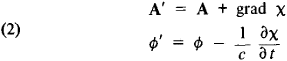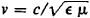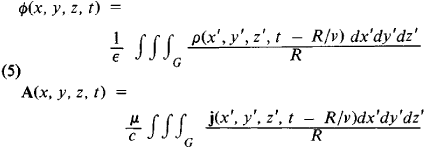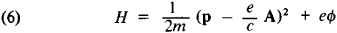# Potentials, Electromagnetic Field

## Potentials, Electromagnetic Field

quantities characterizing an electromagnetic field. In electrostatics, a vector electric field may be characterized by a single scalar function —the electrostatic potential. In general, to describe any arbitrary electromagnetic field, two quantities, namely the vector potential A(x, y, z, t) and the scalar potential ϕ (ξ, y, z, t), where x, y, and z are coordinates and t is the time, may be introduced in place of the two vectors magnetic induction B and electric field intensity E. Here, B and E are uniquely determined in terms of A and ϕ:where c is the speed of light in a vacuum.

The equations for the field potentials are simpler in form than those derived from Maxwell’s basic equations, and electromagnetic field potentials therefore simplify the problem of finding the variables of electromagnetic fields. The simplification occurs in that the potentials are not uniquely determined. If we introduce in place of B and ϕ the new potentialswhere x is an arbitrary function of the coordinates and time, then vectors B and E defined by equations (1) will not change. The invariance of the electromagnetic field with respect to the transformations of potentials given by equations (2) is called gauge invariance. Gauge invariance permits an additional restriction on the electromagnetic field potentials, usually through the Lorentz conditionwhere ∈ and μ are the dielectric constant and magnetic permeability of the medium. When condition (3) is imposed, the equations for electromagnetic field potentials obtained from Maxwell’s equations take on identical form in a homogeneous medium (∈ = const and μ = const):Here, Δ is the Laplace operator, ρ and j are the charge density and current density, andis the rate of propagation of the electromagnetic field in the medium. If ρ = 0 and j = 0, then the electromagnetic field potentials satisfy the wave equations.

Equations (4) permit the determination of the potentials A and ϕ from a known distribution of charges and currents, and through equations (1), of B and E of the electromagnetic field. Individual solutions of equations (4) that satisfy the principle of causality are called retarded potentials. The retarded potentials at a point with coordinates x, y, and ζ at time t are determined by the charge and current densities at a point with coordinates x″, y″, and z″ at a previous time τ = t — R/ν, whereis the distance from the source point to the point of observation.

If the charges and currents are distributed in a finite region of space G, then the retarded potentials are determined by summing, or integrating, the elementary potentials from the charges and currents concentrated in infinitesimal volumes dx″dy″dz″, with consideration for the delay time:The Hamiltonian function H of a charged particle moving in an electromagnetic field is expressed in terms of the electromagnetic field potentials:where ρ is the momentum of the particle and e and m are its charge and mass. In quantum mechanics the Hamiltonian operator is also expressed in terms of electromagnetic field potentials.

G. IA. MIAKISHEV

Site: Follow: Share:
Open / Close